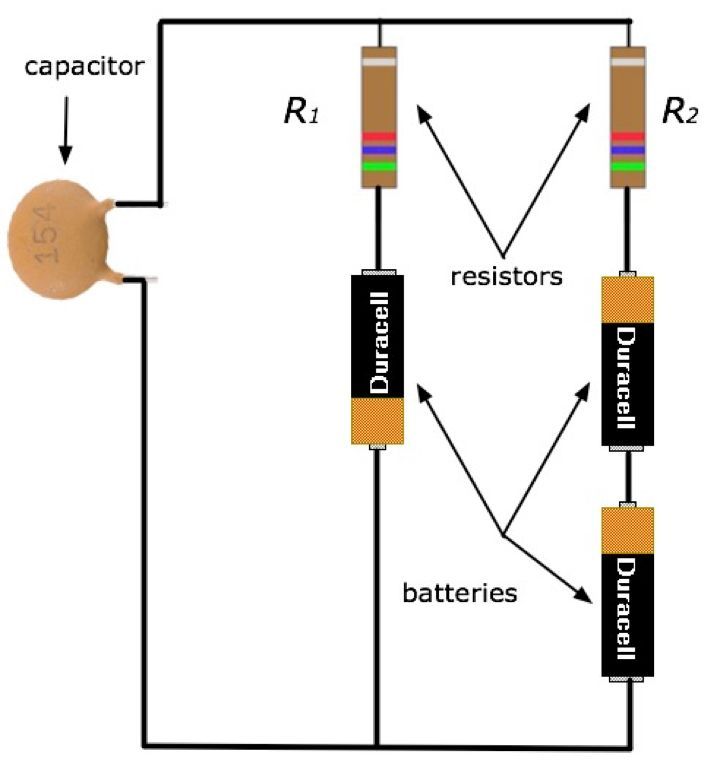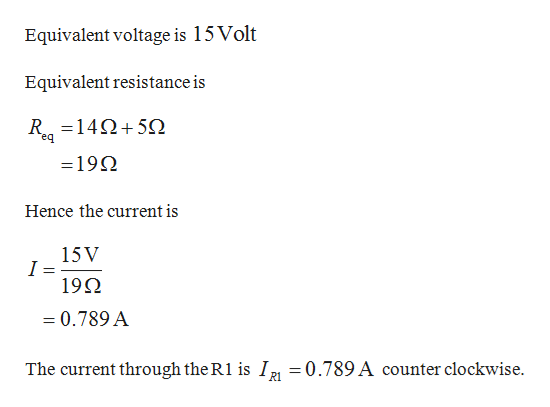# capacitorR1R2resistorsbatteries154DuracellDuracellDuracell

Question
6 views
 In the figure is shown another circuit that can be used to model a cell membrane. In this particular model, the plates of the capacitor (inside the plastic shell shown in the picture) represent the inside and outside of the membrane while the batteries represent different ion channels within the membrane. The opening of different ion channels is modeled by varying the resistances; these changes in the resistance then cause a change in the potential difference between the capacitor plates. For this problem, we will only consider fixed values for the resistances. All of the questions below refer to the circuit in its "steady state", meaning that the charge on the capacitor is constant and neither increasing nor decreasing.For each battery, use a potential difference of 5 V (the orange end is the high potential end). Resistor 1 is 14 Ω and resistor 2 is 5 Ω. The capacitor has a capacitance of 0.16 F.

4. What is the potential difference (magnitude) across the capacitor?
VC| = _____ V

5. What is the charge (magnitude) stored on each of the capacitor plates?
|Q| = ______ Chelp_outlineImage Transcriptionclosecapacitor R1 R2 resistors batteries 154 Duracell Duracell Duracell fullscreen
check_circle

Step 1

As per the conditions given the circuit is in steady state so that no current flow through the branch containing capacitor. All batteries are connected in series.help_outlineImage TranscriptioncloseEquivalent voltage is 15Volt Equivalent resistance is R.-14Ω+ 5Ω eq =19 Hence the current is 15V 192 0.789 A The current through the R1 is I2 = 0.789 A counter clockwise. R1 fullscreen
Step 2

The potential difference acr...

### Want to see the full answer?

See Solution

#### Want to see this answer and more?

Solutions are written by subject experts who are available 24/7. Questions are typically answered within 1 hour.*

See Solution
*Response times may vary by subject and question.
Tagged in

### Current Electricity